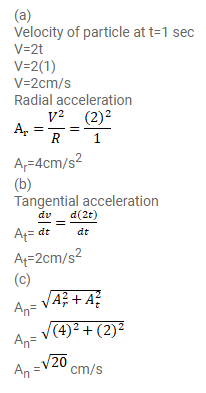# A particle moves in a circle of radius

Question:

A particle moves in a circle of radius $1.0 \mathrm{~cm}$ at a speed given by $\mathrm{v}=2.0 \mathrm{t}$ where $\mathrm{v}$ is in $\mathrm{cm} / \mathrm{s}$ and $t$ in seconds.

(a) Find the radial acceleration of the particle at $t=1 \mathrm{~s}$.

(b) Find the tangential acceleration at $t=1 \mathrm{~s}$.

(c) Find the magnitude of the acceleration at $\mathrm{t}=1 \mathrm{~s}$.

Solution: